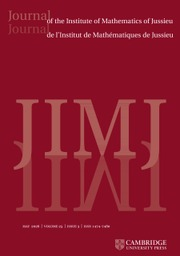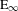Home
Hostname: page-component-cf9d5c678-cnwzk Total loading time: 0.432 Render date: 2021-08-02T23:07:26.941Z Has data issue: true Feature Flags: { "shouldUseShareProductTool": true, "shouldUseHypothesis": true, "isUnsiloEnabled": true, "metricsAbstractViews": false, "figures": true, "newCiteModal": false, "newCitedByModal": true, "newEcommerce": true, "newUsageEvents": true }Journal of the Institute of Mathematics of Jussieu

## Abstract

This paper sets up the foundations for derived algebraic geometry, Goerss–Hopkins obstruction theory, and the construction of commutative ring spectra in the abstract setting of operadic algebras in symmetric spectra in an (essentially) arbitrary model category. We show that one can do derived algebraic geometry a la Toën–Vezzosi in an abstract category of spectra. We also answer in the affirmative a question of Goerss and Hopkins by showing that the obstruction theory for operadic algebras in spectra can be done in the generality of spectra in an (essentially) arbitrary model category. We construct strictly commutative simplicial ring spectra representing a given cohomology theory and illustrate this with a strictly commutative motivic ring spectrum representing higher order products on Deligne cohomology. These results are obtained by first establishing Smith’s stable positive model structure for abstract spectra and then showing that this category of spectra possesses excellent model-theoretic properties: we show that all colored symmetric operads in symmetric spectra valued in a symmetric monoidal model category are admissible, i.e., algebras over such operads carry a model structure. This generalizes the known model structures on commutative ring spectra and$\text{E}_{\infty }$-ring spectra in simplicial sets or motivic spaces. We also show that any weak equivalence of operads in spectra gives rise to a Quillen equivalence of their categories of algebras. For example, this extends the familiar strictification of$\text{E}_{\infty }$-rings to commutative rings in a broad class of spectra, including motivic spectra. We finally show that operadic algebras in Quillen equivalent categories of spectra are again Quillen equivalent. This paper is also available at arXiv:1410.5699v2.

Type
Research Article
Information

## Access options

Get access to the full version of this content by using one of the access options below. (Log in options will check for institutional or personal access. Content may require purchase if you do not have access.)

## Footnotes

The original version of this article was published with some references containing links to pirated websites. A notice detailing with this has been published and the error rectified in the online PDF and HTML copies.

## References

Aguiar, M. and Mahajan, S., Monoidal Functors, Species and Hopf Algebras, CRM Monograph Series, Volume 29 (American Mathematical Society, Providence, RI, 2010), http://math.tamu.edu/∼maguiar/a.pdf.CrossRefGoogle Scholar
Barwick, C., On left and right model categories and left and right Bousfield localizations, Homology Homotopy Appl. 12(2) (2010), 245320, arXiv:0708.2067.CrossRefGoogle Scholar
Batanin, M. and Berger, C., Homotopy theory for algebras over polynomial monads, Preprint, 2013, arXiv:1305.0086v6.Google Scholar
Behrend, K., Differential graded schemes I: perfect resolving algebras, Preprint, 2002, arXiv:math/0212225v1.Google Scholar
Beĭlinson, A. A., Higher Regulators and Values of L-Functions, Current Problems in Mathematics, Volume 24, pp. 181238 (Itogi Nauki i Tekhniki, vol. 30, Akad. Nauk SSSR, Vsesoyuz. Inst. Nauchn. i Tekhn. Inform, Moscow, 1984), http://math.stanford.edu/∼conrad/BSDseminar/refs/BeilinsonConj.pdf.Google Scholar
Bayeh, M., Hess, K., Karpova, V., Kȩdziorek, M., Riehl, E. and Shipley, B., Left-induced model structures and diagram categories, in Women in Topology: Collaborations in Homotopy Theory, Contemporary Mathematics, Volume 641, pp. 4981 (American Mathematical Society, Providence, RI, 2015), arXiv:1401.3651v2.CrossRefGoogle Scholar
Berger, C. and Moerdijk, I., Axiomatic homotopy theory for operads, Comment. Math. Helv. 78(4) (2003), 805831, arXiv:math/0206094.CrossRefGoogle Scholar
Berger, C. and Moerdijk, I., On the derived category of an algebra over an operad, Georgian Math. J. 16(1) (2009), 1328, arXiv:0801.2664v2.Google Scholar
Borceux, F., Handbook of Categorical Algebra. 1, Encyclopedia of Mathematics and its Applications, Volume 50 (Cambridge University Press, Cambridge, 1994).Google Scholar
Cisinski, D.-C. and Déglise, F., Triangulated categories of mixed motives, Preprint, 2009, arXiv:0912.2110v3.Google Scholar
Deninger, C., Higher order operations in Deligne cohomology, Invent. Math. 120(2) (1995), 289315. https://wwwmath.uni-muenster.de/u/weckerm/deninger/about/publikat/cd25.ps.CrossRefGoogle Scholar
Elmendorf, A. D. and Mandell, M. A., Rings, modules, and algebras in infinite loop space theory, Adv. Math. 205(1) (2006), 163228, arXiv:math/0403403.CrossRefGoogle Scholar
Esnault, H. and Viehweg, E., Deligne–Beĭlinson Cohomology, Beĭlinson’s Conjectures on Special Values of L-Functions, Perspect. Math., Volume 4, pp. 4391 (Academic Press, Boston, MA, 1988), http://mi.fu-berlin.de/users/esnault/preprints/ec/deligne_beilinson.pdf.CrossRefGoogle Scholar
Fresse, B., Modules Over Operads and Functors, Lecture Notes in Mathematics, vol. 1967 (Springer, Berlin, 2009), arXiv:0704.3090v4.CrossRefGoogle Scholar
Gorchinskiy, S. and Guletskii, V., Positive model structures for abstract symmetric spectra, Preprint, 2011. arXiv:1108.3509v3http://dx.doi.org/10.1007/s10485-016-9480-9.CrossRefGoogle Scholar
Gorchinskiy, S. and Guletskiĭ, V., Symmetric powers in abstract homotopy categories, Adv. Math. 292 (2016), 707754, arXiv:0907.0730v4.CrossRefGoogle Scholar
Goerss, P. G. and Hopkins, M. J., Moduli problems for structured ring spectra (June 8, 2005), http://math.northwestern.edu/∼pgoerss/spectra/obstruct.pdf.Google Scholar
Goerss, P. G. and Hopkins, M. J., Moduli Spaces of Commutative Ring Spectra, Structured Ring Spectra, London Mathematical Society Lecture Note Series, Volume 315, pp. 151200 (Cambridge University Press, Cambridge, 2004), http://math.northwestern.edu/∼pgoerss/papers/sum.pdf.CrossRefGoogle Scholar
Harper, J. E., Homotopy theory of modules over operads in symmetric spectra, Algebr. Geom. Topol. 9(3) (2009), 16371680, arXiv:0801.0193v3.CrossRefGoogle Scholar
Harper, J. E., Homotopy theory of modules over operads and non-𝛴 operads in monoidal model categories, J. Pure Appl. Algebra 214(8) (2010), 14071434, arXiv:0801.0191.CrossRefGoogle Scholar
Hinich, V., Homological algebra of homotopy algebras, Comm. Algebra 25(10) (1997), 32913323, arXiv:q-alg/9702015.CrossRefGoogle Scholar
Hirschhorn, P. S., Model Categories and Their Localizations, Mathematical Surveys and Monographs, Volume 99 (American Mathematical Society, Providence, RI, 2003).Google Scholar
Hornbostel, J., Preorientations of the derived motivic multiplicative group, Algebr. Geom. Topol. 13(5) (2013), 26672712, arXiv:1005.4546.CrossRefGoogle Scholar
Hovey, M., Model Categories, Mathematical Surveys and Monographs, Volume 63 (American Mathematical Society, Providence, RI, 1999).Google Scholar
Hovey, M., Spectra and symmetric spectra in general model categories, J. Pure Appl. Algebra 165(1) (2001), 63127, arXiv:math/0004051.CrossRefGoogle Scholar
Hopkins, M. J. and Quick, G., Hodge filtered complex bordism, J. Topol. 8(1) (2015), 147183, arXiv:1212.2173v3.CrossRefGoogle Scholar
Hess, K. and Shipley, B., Waldhausen K-theory of spaces via comodules, Preprint, 2014. arXiv:1402.4719v2, http://dx.doi.org/10.1016/j.aim.2015.12.019.CrossRefGoogle Scholar
Holmstrom, A. and Scholbach, J., Arakelov motivic cohomology I, J. Algebraic Geom. 24(4) (2015), 719754, arXiv:1012.2523.CrossRefGoogle Scholar
Hovey, M., Shipley, B. and Smith, J., Symmetric spectra, J. Amer. Math. Soc. 13(1) (2000), 149208, arXiv:math/9801077.CrossRefGoogle Scholar
Jardine, J. F., Motivic symmetric spectra, Doc. Math. 5 (2000), 445553.Google Scholar
Lurie, J., Higher algebra (September 18, 2017), http://math.harvard.edu/∼lurie/papers/HA.pdf.Google Scholar
Lurie, J., Higher Topos Theory, Annals of Mathematics Studies, Volume 170 (Princeton University Press, Princeton, NJ, 2009), http://math.harvard.edu/∼lurie/papers/HTT.pdf.CrossRefGoogle Scholar
Mandell, M. A., May, J. P., Schwede, S. and Shipley, B., Model categories of diagram spectra, Proc. Lond. Math. Soc. (3) 82(2) (2001), 441512, http://www.math.uiuc.edu/K-theory/0320/.CrossRefGoogle Scholar
Pereira, L. A., Cofibrancy of operadic constructions in positive symmetric spectra, Preprint, 2014, arXiv:1410.4816v2, http://dx.doi.org/10.4310/hha.2016.v18.n2.a7.CrossRefGoogle Scholar
Pavlov, D. and Scholbach, J., Admissibility and rectification of colored symmetric operads, J. Topol. (to appear), Preprint, 2014, arXiv:1410.5675v3, https://doi.org/10.1112/topo.12008.CrossRefGoogle Scholar
Pavlov, D. and Scholbach, J., Homotopy theory of symmetric powers, Homology, Homotopy Appl. 20(1) (2018), 359397, arXiv:1510.04969v3, http://dx.doi.org/10.4310/HHA.2018.v20.n1.a20.CrossRefGoogle Scholar
Richter, B., Symmetry properties of the Dold–Kan correspondence, Math. Proc. Cambridge Philos. Soc. 134(1) (2003), 95102, http://www.math.uni-hamburg.de/home/richter/doldkan.pdf.CrossRefGoogle Scholar
Saito, M., Mixed Hodge modules and applications, in Proceedings of the International Congress of Mathematicians, Volumes I, II (Kyoto 1990), pp. 725734 (Mathematical Society, Japan, Tokyo, 1991), http://mathunion.org/ICM/ICM1990.1/Main/icm1990.1.0725.0734.ocr.pdf.Google Scholar
Scholbach, J., Special L-values of geometric motives, Asian Journal of Mathematics 21(2) (2017), 225264, arXiv:1003.1215, http://dx.doi.org/10.4310/AJM.2017.v21.n2.a2.CrossRefGoogle Scholar
Schwede, S., Spectra in model categories and applications to the algebraic cotangent complex, J. Pure Appl. Algebra 120(1) (1997), 77104, http://people.math.uni-bonn.de/schwede/modelspec.pdf.CrossRefGoogle Scholar
Schreiber, U., Differential cohomology in a cohesive infinity-topos, Preprint, 2013, arXiv:1310.7930v1.Google Scholar
Shipley, B., A convenient model category for commutative ring spectra, in Homotopy Theory: Relations With Algebraic Geometry, Group Cohomology, and Algebraic K-Theory, Contemporary Mathematics, Volume 346, pp. 473483 (American Mathematical Society, Providence, RI, 2004), http://www.math.uic.edu/∼bshipley/com4.pdf.Google Scholar
Shipley, B., Hℤ-algebra spectra are differential graded algebras, Amer. J. Math. 129(2) (2007), 351379, arXiv:math/0209215.CrossRefGoogle Scholar
Spitzweck, M., Operads, algebras and modules in model categories and motives, Bonn: Univ. Bonn. Mathematisch-Naturwissenschaftliche Fakultät (Dissertation), 2001, http://d-nb.info/970107374/34.Google Scholar
Schwede, S. and Shipley, B. E., Algebras and modules in monoidal model categories, Proc. Lond. Math. Soc. (3) 80(2) (2000), 491511, arXiv:math/9801082.CrossRefGoogle Scholar
Schwede, S. and Shipley, B., Equivalences of monoidal model categories, Algebr. Geom. Topol. 3 (2003), 287334, arXiv:math/0209342.CrossRefGoogle Scholar
Schwede, S. and Shipley, B., Stable model categories are categories of modules, Topology 42(1) (2003), 103153, arXiv:math/0108143.CrossRefGoogle Scholar
Sagave, S. and Schlichtkrull, C., Diagram spaces and symmetric spectra, Adv. Math. 231(3–4) (2012), 21162193, arXiv:1103.2764.CrossRefGoogle Scholar
Toën, B. and Vezzosi, G., Homotopical algebraic geometry. II. Geometric stacks and applications, Mem. Amer. Math. Soc. 193(902) (2008), x+224, arXiv:math/0404373.Google Scholar
White, D., Model structures on commutative monoids in general model categories, Preprint, 2014, arXiv:1403.6759v2, http://dx.doi.org/10.1016/j.jpaa.2017.03.001.CrossRefGoogle Scholar
White, D., Monoidal Bousfield localizations and algebras over operads, Preprint, 2014, arXiv:1404.5197v1.Google Scholar

6
Cited by

# Send article to Kindle

Note you can select to send to either the @free.kindle.com or @kindle.com variations. ‘@free.kindle.com’ emails are free but can only be sent to your device when it is connected to wi-fi. ‘@kindle.com’ emails can be delivered even when you are not connected to wi-fi, but note that service fees apply.

Find out more about the Kindle Personal Document Service.

SYMMETRIC OPERADS IN ABSTRACT SYMMETRIC SPECTRA
Available formats
×

# Send article to Dropbox

To send this article to your Dropbox account, please select one or more formats and confirm that you agree to abide by our usage policies. If this is the first time you use this feature, you will be asked to authorise Cambridge Core to connect with your <service> account. Find out more about sending content to Dropbox.

SYMMETRIC OPERADS IN ABSTRACT SYMMETRIC SPECTRA
Available formats
×

# Send article to Google Drive

To send this article to your Google Drive account, please select one or more formats and confirm that you agree to abide by our usage policies. If this is the first time you use this feature, you will be asked to authorise Cambridge Core to connect with your <service> account. Find out more about sending content to Google Drive.

SYMMETRIC OPERADS IN ABSTRACT SYMMETRIC SPECTRA
Available formats
×
×